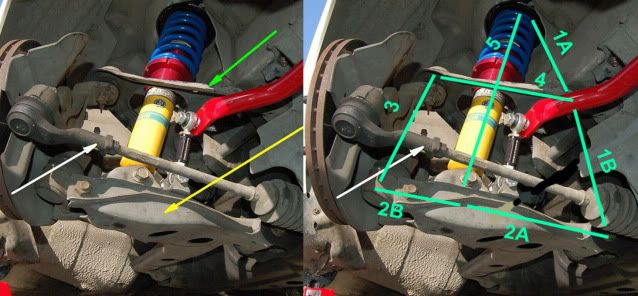1990 Mazda Miata Suspension

# Alex Uchida

## IntroductionThe suspension pictured above is from a 1990 Mazda Miata. The Miata suspension design uses a common 4 link suspension to provide great handling performance. In this project I used the position, velocity, and acceleration from the input force to calculate the bearing reaction forces on the suspension components.

## Input Force

The input force is provided by the surface of the road profile. Using a cosine wave to simulate the surface of the road, the angle theta 2 can be used as the input to the system.

#### Calculating the Input

(1)
\begin{align} \theta_2 = \frac{A\cos(\omega t)}{r_2_a + r_2_b} \end{align}
(2)
\begin{align} \frac{d\theta_2}{d\omega} = -\frac{A\omega\sin(\omega t)}{r_2_a + r_2_b} \end{align}
(3)
\begin{align} \frac{d\theta_2^2}{d^2\omega} = -\frac{A\omega^2\cos(\omega t)}{r_2_a + r_2_b} \end{align}

## Position

Solving the loop equations in MATLAB provides the unknown link lengths and angles.

#### Solving for unknowns

(4)
\begin{equation} r_5 = -r_1_a -r_1_b -r_2_a \end{equation}
(5)
\begin{align} \theta_5 = \tan^-^1\frac{r_5_y}{r_5_x} \end{align}
(6)
\begin{equation} -r_4 = r_1_b + r_2_a + r_2_b + r_3 \end{equation}
(7)
\begin{align} \theta_4 = \tan^-^1\frac{r_4_y}{r_4_x} \end{align}

## Velocity and Acceleration Analysis

Taking the first derivative of the loop equations with respect to time produces two new loop equations that can be solved to find the velocity at each link. Taking the second derivative of the loop equations with respect to time produces two new loop equations that can be solved for the acceleration of each link. The matrices corresponding to velocity and acceleration are shown below in the MATLAB code.

## MATLAB Code

clear all %Alex Uchida
dtr = pi/180;

r2a = 8.25;
r2b = 5;
r3 = 8.5;
r4 = 4.73; % calculated from matlab
r5 = 15.44; % calculated from matlab
r34 = 4.25;
r32 = 4.25;

% Solving for Input(theta2) from road profile
amp = 2; % amplitude
L = 11; % distance between bumps (ft)
v = 30; % velocity (mph)
V = (v*5280)/3600; % velocity (ft/s)
W = (V/L)*2*pi;

theta2 = (amp*cos(W))/(13.5*dtr);
theta2_dot = -(amp*W*sin(W))/(13.5);
theta2_ddot = (amp*W*W*cos(W))/13.5;

% angles
theta3 = 90*dtr;
theta4 = 33.46*dtr; %from matlab
theta5 = 87.88*dtr; %from matlab

% assumptions
m2 = 5; % arm mass (lb)
m3 = 50; % wheel mass (lb)
ig3 = 52; % 1/12(b)(r3^3)
ig2 = 205; % 1/12(b)(r2a+rsb)^3
grav = 387.24; % in/s^2
ag2x = .01;
ag3x = .001;

%make the matrix easier
s2 = sin(theta2);
c2 = cos(theta2);
s3 = sin(theta3);
c3 = cos(theta3);
s4 = sin(theta4);
c4 = cos(theta4);
s5 = sin(theta5);
c5 = cos(theta5);

%solving for velocity
A = [0, 0, -r5*s5, c5;
0, 0, r5*c5, s5;
-r3*s3, -r4*s4, 0, 0;
r3*c3, r4*c4, 0, 0];

b = [r2a*theta2_dot*s2; -r2a*theta2_dot*c2; (r2a*theta2_dot*s2) + (r2b*theta2_dot*s2); (-r2a*theta2_dot*c2) - (r2b*theta2_dot*c2)];

v = inv(A)*b;

theta3_dot = v(1);
theta4_dot = v(2);
theta5_dot = v(3);
r5_dot = v(4);

%solving for acceleration
c = [((r2a*theta2_ddot*s2) + (r2a*theta2_dot*theta2_dot*c2) + (r5*theta5_dot*theta5_dot*c5) + (2*r5_dot*theta5_dot*s5));
((-r2a*theta2_ddot*c2) + (r2a*theta2_dot*theta2_dot*s2) + (r5*theta5_dot*theta5_dot*s5) - (2*r5_dot*theta5_dot*c5));
((r2a*theta2_ddot*s2) + (r2a*theta2_dot*theta2_dot*c2) + (r2b*theta2_ddot*s2) + (r2b*theta2_dot*theta2_dot*c2) + (r3*theta3_dot*theta3_dot*c3) + (r4*theta4_dot*theta4_dot*c4));
((-r2a*theta2_ddot*c2) + (r2a*theta2_dot*theta2_dot*s2) - (r2b*theta2_ddot*c2) + (r2b*theta2_dot*theta2_dot*s2) + (r3*theta3_dot*theta3_dot*c3) + (r4*theta4_dot*theta4_dot*s4))];

a = inv(A)*c;

theta3_ddot = a(1);
theta4_ddot = a(2);
theta5_ddot = a(3);
r5_ddot = a(4);

%solving for bearing reaction forces

B = [1, 1, 0, 0, 0, 0, 0, 0, 0, 0, 0, 0;
0, 0, 1, 1, 0, 0, 0, 0, 0, 0, 0, 0;
0, 0, 0, 0, c4, 1, 0, 0, 0, 0, 0, 0;
0, 0, 0, 0, s4, 0, 1, 0, 0, 0, 0, 0;
0, 0, 0, 0, (r34*c3*s4 - r34*s3*c4), -r32*sin(theta3+pi), r32*cos(theta3+pi), 0, 0, 0, 0, 0;
0, 0, 0, 0, 0, 0, 0, 1, 0, 1, 0, (c5+c2);
0, 0, 0, 0, 0, 0, 0, 0, 1, 0, 1, (s5+s2);
0, 0, 0, 0, 0, 0, 0, -r2b*s2, r2b*c2, -r2a*s2, r2a*c2, 1;
1, 0, 0, 0, 1, 0, 0, 0, 0, 0, 0, 0;
0, 0, 1, 0, 0, 0, 0, 0, 0, 0, 0, 1;
0, 0, 0, 0, 0, 1, 0, 1, 0, 0, 0, 0;
0, 0, 0, 0, 0, 0, 1, 0, 1, 0, 0, 0];

d = [0; 0; m3*ag3x; m3*grav; ig3*theta3_ddot; m2*ag2x; m2*grav; (ig2*theta2_ddot +100); 0; 0; 0; 0];

F = inv(B)*d;

%all units for force are in psi

F43 = F(1)
F41 = F(2)
F52 = F(3)
F51 = F(4)
F34 = F(5)
F32x = F(6)
F32y = F(7)
F23x = F(8)
F23y = F(9)
F21x = F(10)
F21y = F(11)
F25 = F(12)

page revision: 2, last edited: 15 Dec 2010 00:26